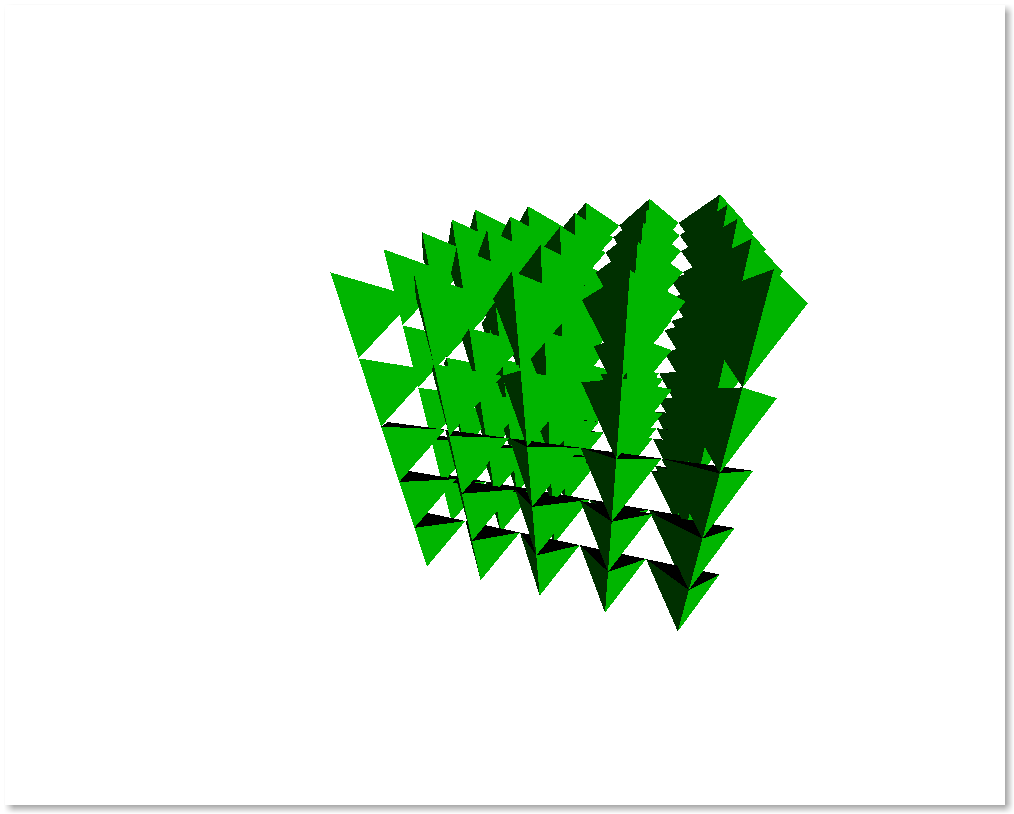# Graphics and Visualization¶

## Intro¶

The chemlab.graphics package is one of the most interesting aspects of chemlab, that sets him apart from similar programs.

The purpose of the package is to provide a solid library to develop 3D applications to display chemical data in an flexible way. For example it’s extremely easy to build a molecular viewer and add a bunch of custom features to it.

The typical approach when developing a graphics application is to create a QtViewer instance and add 3D features to it:

>>> from chemlab.graphics import QtViewer
>>> v = QtViewer()


now let’s define a molecule. We can use the chemlab.db module to get a water template.

>>> from chemlab.graphics.renderers import AtomRenderer
>>> from chemlab.db  import ChemlabDB
>>> water = ChemlabDB().get('molecule', 'example.water')
>>> ar = v.add_renderer(AtomRenderer, water.r_array, water.type_array)
>>> v.run()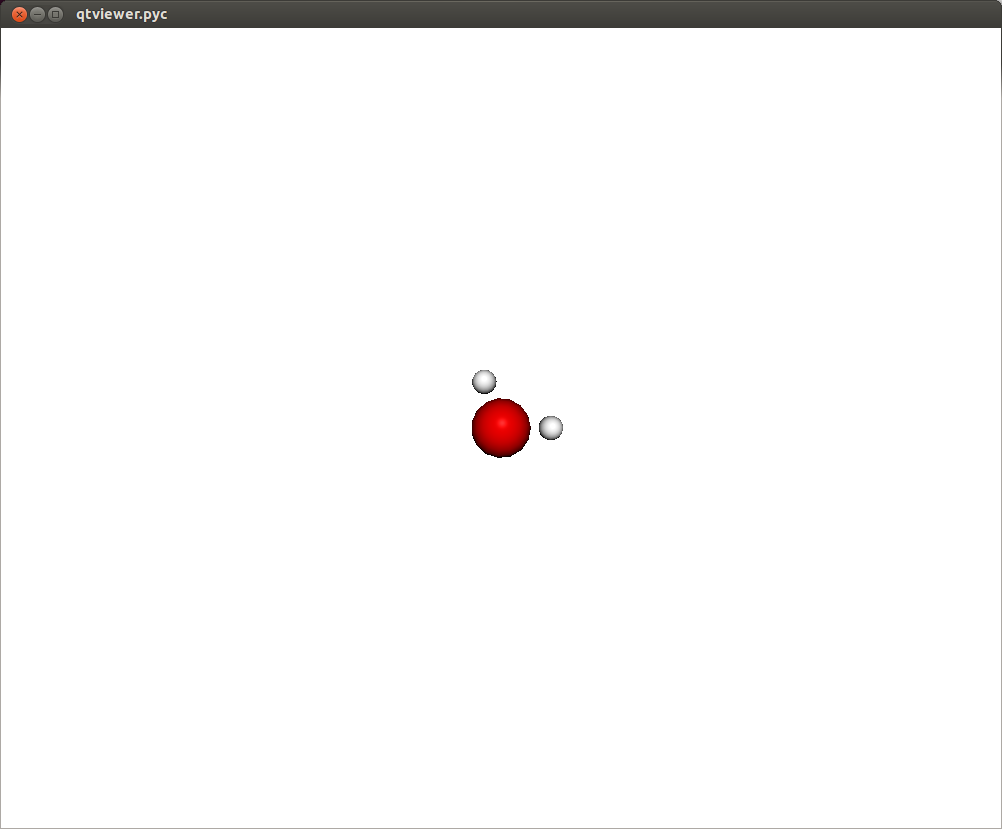In this way you should be able to visualize a molecule where each atom is represented as a sphere. There are also a set of viewing controls:

• Mouse Drag (Left Click) or Left/Right/Up/Down: Rotate the molecule
• Mouse Drag (Right Click): Pan the view
• Mouse Wheel or +/-: Zoom in/out

In a similar fashion it is possible to display other features, such as boxes, cylinders, lines, etc. It is useful to notice that with Viewer.add_renderer we are not passing an instance of the renderer, but we’re passing the renderer class and its respective constructor arguments. The method Viewer.add_renderer returns the actual instance.

It is possible as well to overlay 2D elements to a scene in a similar fashion, this will display a string at the screen position 300, 300:

from chemlab.graphics.uis import TextUI
tui = v.add_ui(TextUI, 300, 300, "Hello, World!")


Anyway, I encourage you to use the powerful Qt framework to provide interaction and widgets to your application.

## Renderers¶

Renderers are simply classes used to draw 3D objects. They are tecnically required to provide just one method, draw and they must take an instance of QChemlabWidget as their first argument (check out the AbstractRenderer class). In this way they provide the maximum flexibility required to build efficient opengl routines. Renderers may be subclass other renderers as well as use other renderers.

A very useful renderer is TriangleRenderer, used to render efficiently a list of triangles, it constitutes a basis for writing other renderers. TriangleRenderer works like this: you pass the vertices, normals and colors of the triangles and it will display a set of triangles in the world:

from chemlab.graphics import QtViewer
from chemlab.graphics.renderers import TriangleRenderer
from chemlab.graphics.colors import green
import numpy as np

vertices = np.array([[0.0, 0.0, 0.0], [0.0, 1.0, 0.0], [1.0, 0.0, 0.0]])
normals = np.array([[0.0, 0.0, 1.0], [0.0, 0.0, 1.0], [0.0, 0.0, 1.0]])
colors = np.array([green, green, green])

v = QtViewer()
v.run()If you pass 6 vertices/normals/colors, it will display 2 triangles and so on. As a sidenote, it is very efficient and in fact chemlab.graphics.renderers.TriangleRenderer is used as a backend for a lot of other renderers such as SphereRenderer and CylinderRenderer. If you can reduce a shape in triangles, you can easily write a renderer for it.

In addition to that, TriangleRenderer provides also a method to update vertices, normals and colors. We can demonstrate that from the last example by defining an update function that rotates our triangle:

from chemlab.graphics.transformations import rotation_matrix

def update():
y_axis = np.array([0.0, 1.0, 0.0])

# We take the [:3,:3] part because rotation_matrix can be used to
# rotate homogeneous (4D) coordinates.
rot = rotation_matrix(3.14/32, y_axis)[:3, :3]

# This is the numpy-efficient way of applying rot to each coordinate
vertices[:] = np.dot(vertices, rot.T)
normals[:] = np.dot(vertices, rot.T)

tr.update_vertices(vertices)
tr.update_normals(normals)
v.widget.update()

v.schedule(update, 10)
v.run()


## Post Processing Effects¶

New in version 0.3.

Post processing effects are a great way to increase the visual quality of your representations. Those effects are applied after the scene is rendered and they can be applied one after each other to achieve a combination of effects.

Applying a post processing effect is extremely easy. Let’s see we are viewing a big molecule with lots of pockets, such as a protein. Grab the protein 3ZJE , load it into chemlab and display it using a simple Van der Waals representation:

from chemlab.graphics import QtViewer
from chemlab.graphics.renderers import AtomRenderer
from chemlab.io import datafile

v = QtViewer()
v.run()


You’ll get a representation like this: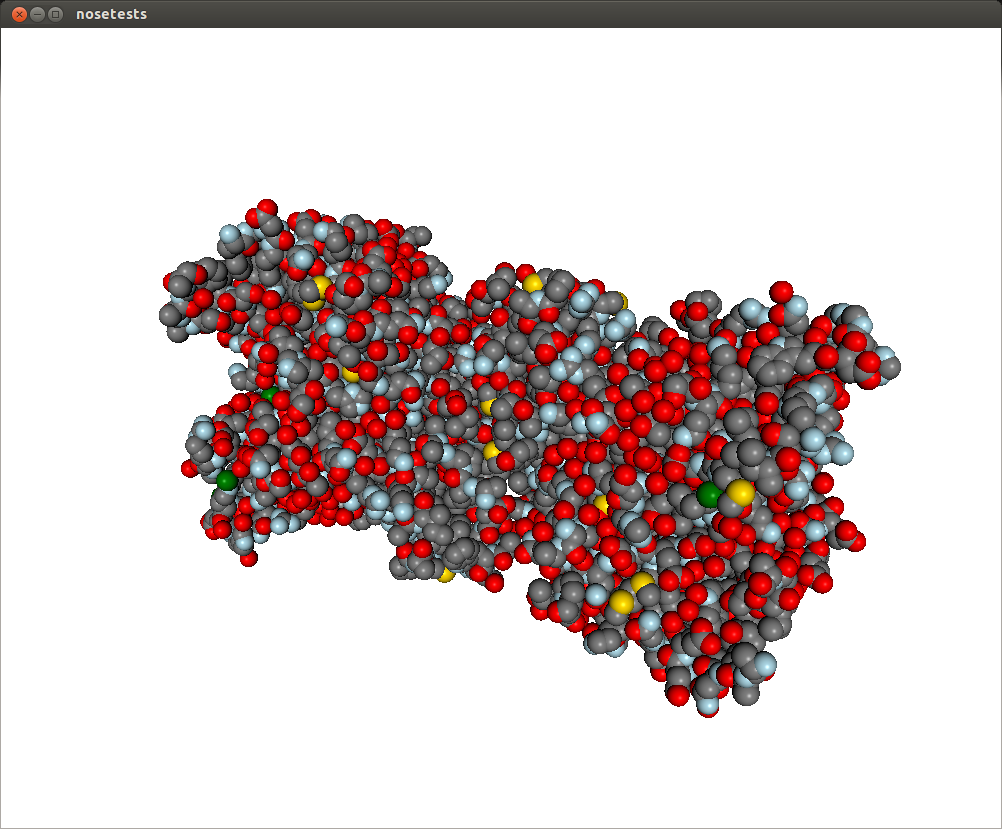This representation doesn’t really show the molecule surface features, plus it looks dull and plasticky. We can add the screen space ambient occlusion effect to improve its visual quality.

Screen space ambient occlusion (SSAO) is a very powerful technique used by numerous videogames to make the illumination much more realistic, by darkening the more occluded areas of the objects, such as pockets and surface features.

Chemlab implements this effect in the SSAOEffect class. To apply it to the scene it’s sufficient to add this simple line:

from chemlab.graphics.postprocessing import SSAOEffect

v.run()


What you’ll get is this, with a much-improved visual quality: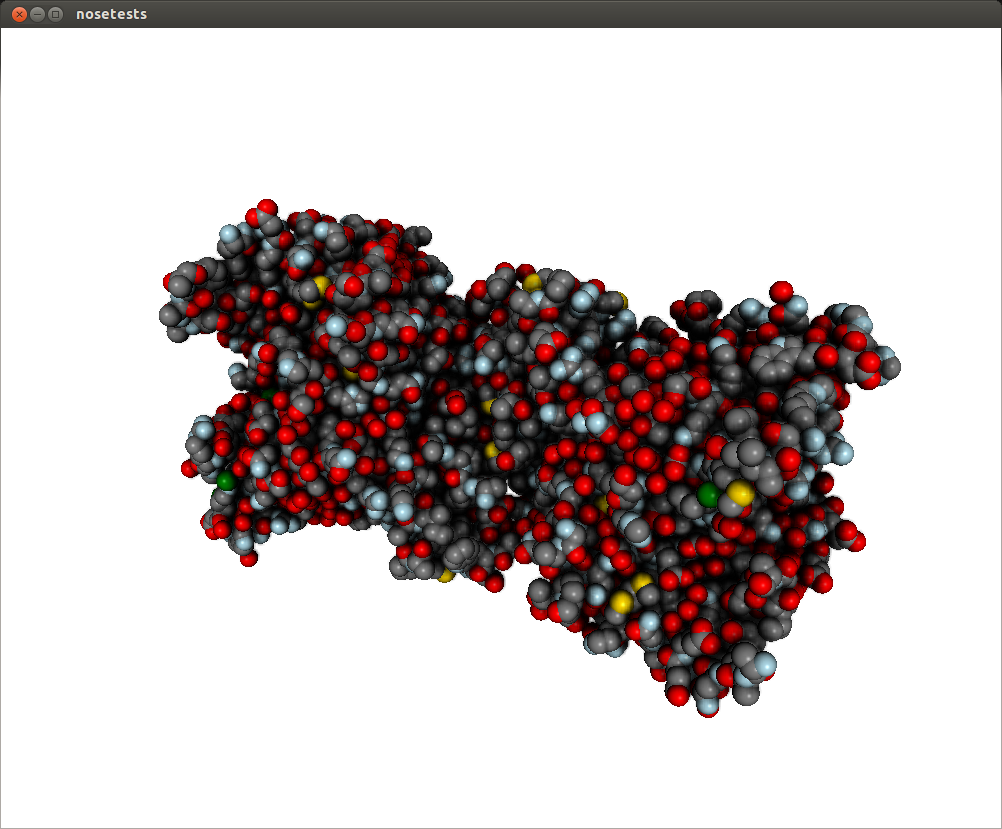Post processing effects can be customized with arguments. The SSAO effect may have a dirty look, you can fix that by changing the parameter kernel_size, which default to 32, with a max value of 128:

v.add_post_processing(SSAOEffect, kernel_size=128)


This will improve the visual quality at the cost of decreased performance. To see all the options available take look at the api documentation List of Post Processing Effects.

Post processing effects can also be stacked on top of each other. If your computer is powerful enough, you can load your scene with a stack of effects that will be applied in turn:

from chemlab.graphics.postprocessing import SSAOEffect
from chemlab.graphics.postprocessing import OutlineEffect
from chemlab.graphics.postprocessing import FXAAEffect
from chemlab.graphics.postprocessing import GammaCorrectionEffect

v.run()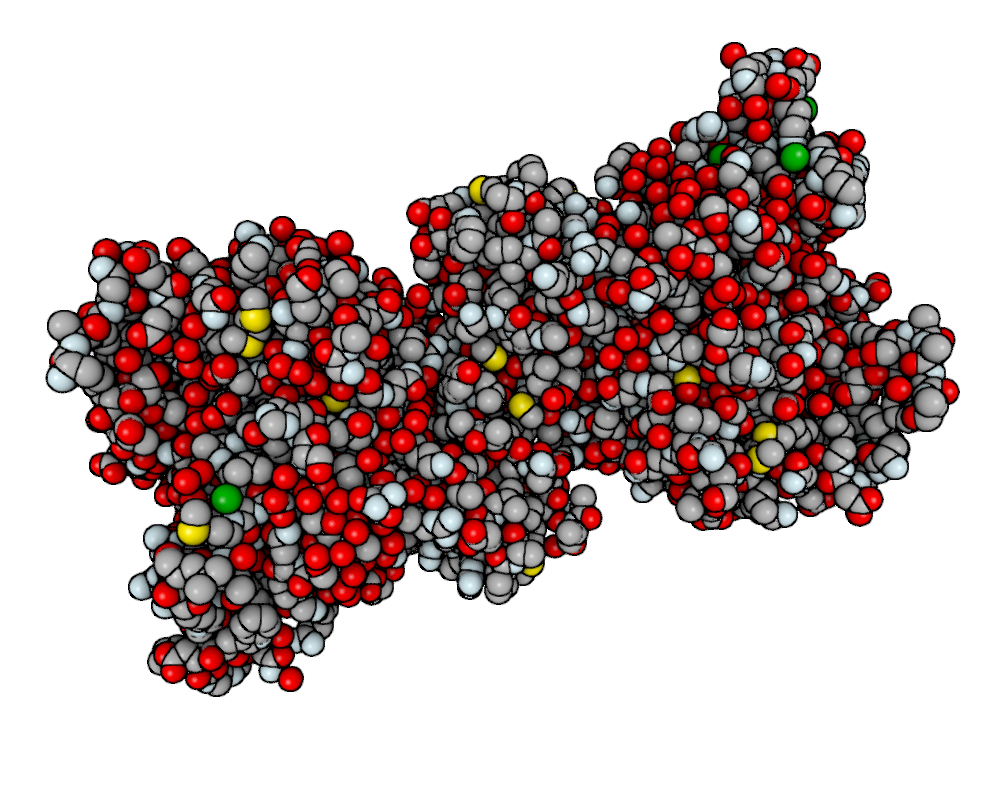Unfortunately on ATI cards with open source drivers can’t apply multiple post processing effects. I’m investigating the issue, but this can be potentially due to a bug in the drivers.

## Offline Rendering¶

New in version 0.3.

With chemlab you can produce renderings programmatically without having to display anything or tinkering with the user interface. This feature comes pretty useful when generating reports with a lot of pictures.

Let’s say you want to make a showcase of different chemical compounds, such as the first four alkanes. First of all we’ll take a sample molecule to adjust the looks and then we’ll adapt the code to render all of the alkanes in a sequence.

As an example we’ll tweak our rendering on the norbornene molecule contained in the chemlab database:

from chemlab.db import ChemlabDB
cdb = ChemlabDB()

norb = cdb.get("molecule", "example.norbornene")


We want to do the rendering of this molecule using a space fill representation, this can be achieved by using the AtomRenderer, which will render each atom as a sphere with its Van Der Waals radius:

from chemlab.graphics import QtViewer
from chemlab.graphics.renderers import AtomRenderer

v = QtViewer()


After we’ve got the renderer in place we can programmatically manipulate the camera to adjust at the right zoom level. You can, for instance, use the chemlab.graphics.camera.Camera.autozoom() method to automatically adjust the scene, but you are free to use any other method present in the Camera class:

v.widget.camera.autozoom(norb.r_array)

v.run()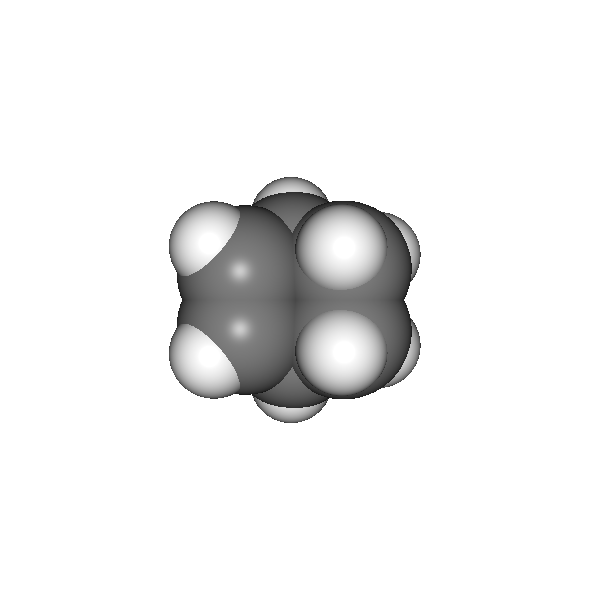At this point, you are free experiment with different effects and combinations. In our case we’ll add SSAO and anti aliasing to add more depth and smoothness to the rendering:

from chemlab.graphics.postprocessing import SSAOEffect, FXAAEffect

v.run()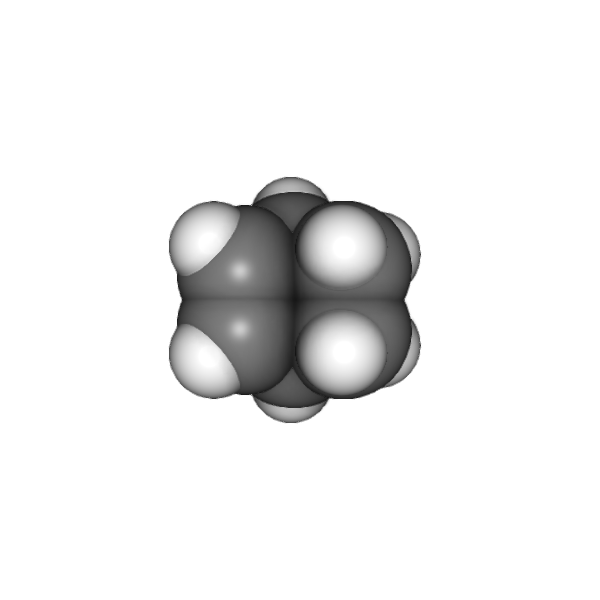To actually save the image you can now use the chemlab.graphics.QChemlabWidget.toimage() method and select a resolution of 800x800 pixels. This will return a PIL image, that has a save method to store it as a png:

img = v.widget.toimage(800, 800)
img.save("norb.png")


Once we’ve got the sample molecule up and running it’s very easy to automatize the process to produce images of different molecules. In the following code we prepare the QtViewer with the effects, we call v.widget.initializeGL() in place of v.show() and for each molecule we add an AtomRenderer and adjust the camera:

from chemlab.db import CirDB
from chemlab.graphics import QtViewer
from chemlab.graphics.renderers import AtomRenderer
from chemlab.graphics.postprocessing import FXAAEffect, SSAOEffect

# A series of compounds to display
compounds = ["methane", "ethane", "propane", "butane"]

db = CirDB()

# Prepare the viewer
v = QtViewer()
v.widget.initalizeGL() # Needed if you don't call show()

for compound in compounds:
mol = db.get("molecule", compound)

v.widget.camera.autozoom(mol.r_array)
# Give some extra zoom
v.widget.camera.mouse_zoom(1.0)

v.widget.toimage(300, 300).save(compound + '.png')

# Cleanup
v.remove_renderer(rend)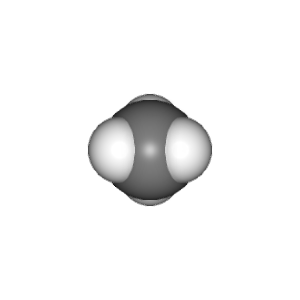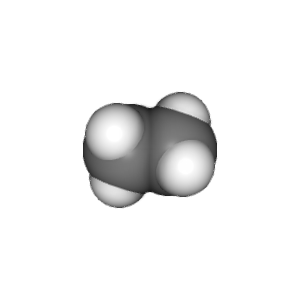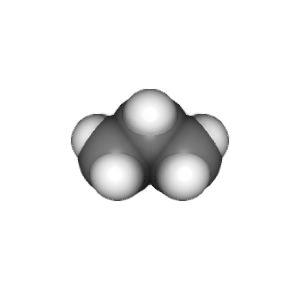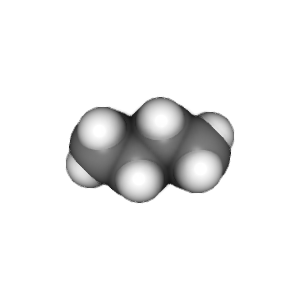This example is stored in the chemlab/examples/offline_rendering.py file.

## Tutorial: TetrahedronRenderer¶

Note

This section is mainly for developers.

In this section, we’ll see how to write a renderer that will display several tetrahedrons. We will write our TetrahedronRenderer based on TriangleRenderer. To do that we first need to understand how a tetrahedron is made, and how can we define the vertices that make the tetrahedron.

First of all, we need to have the 4 coordinates that represents a tetrahedron. Without even trying to visualize it, just pick the values straight from Wikipedia:

import numpy as np
v1 = np.array([1.0, 0.0, -1.0/np.sqrt(2)])
v2 = np.array([-1.0, 0.0, -1.0/np.sqrt(2)])
v3 = np.array([0.0, 1.0, 1.0/np.sqrt(2)])
v4 = np.array([0.0, -1.0, 1.0/np.sqrt(2)])


We can quickly verify if this is correcty by using a PointRenderer:

from chemlab.graphics import QtViewer
from chemlab.graphics.renderers import PointRenderer
from chemlab.graphics.colors import black, green, blue, red

colors = [black, green, blue, red]
v = QtViewer()
v.add_renderer(PointRenderer, np.array([v1, v2, v3, v4]), colors)
v.run()


We’ve got 4 boring points that look like they’re at the vertices of a tetrahedron. Most importantly we learned that we can use PointRenderer to quickly test shapes.

Now let’s define the four triangles (12 vertices) that represent a solid tetrahedron. It is good practice to put the triangle vertices in a certain order to estabilish which face is pointing outside and which one is pointing inside for optimization reasons. The convention is that if we specify 3 triangle vertices in clockwise order this means that the face points outwards from the solid: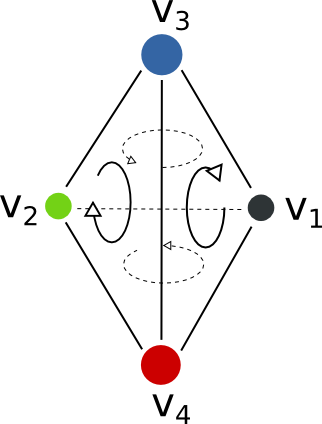We can therefore write our vertices and colors:

vertices = np.array([
v1, v4, v3,
v3, v4, v2,
v1, v3, v2,
v2, v4, v1
])

colors = [green] * 12


All is left to do is write the normals to the surface at each vertex. This is easily done by calculating the cross product of the vectors constituting two sides of a triangle (remember that the normals should point outward) and normalize the result:

n1 = -np.cross(v4 - v1, v3 - v1)
n1 /= np.linalg.norm(n1)

n2 = -np.cross(v4 - v3, v2 - v3)
n2 = np.linalg.norm(n2)

n3 = -np.cross(v3 - v1, v2 - v1)
n3 /= np.linalg.norm(n3)

n4 = -np.cross(v4 - v2, v1 - v2)
n4 /= np.linalg.norm(n4)

normals = [n1, n1, n1,
n2, n2, n2,
n3, n3, n3,
n4, n4, n4]

from chemlab.graphics.renderers import TriangleRenderer

v.run()


Now that we’ve got the basic shape in place we can code the actual Renderer class to be used directly with the viewer. We will make a renderer that, given a set of coordinates will display many tetrahedra.

We can start by defining a Renderer class, inheriting from AbstractRenderer, the main thing you should notice is that you need an additional argument widget that will be passed when you use the method QtViewer.add_renderer:

from chemlab.graphics.renderers import AbstractRenderer

class TetrahedraRenderer(AbstractRenderer):
def __init__(self, widget, positions):
super(TetrahedraRenderer, self).__init__(widget)
...


The strategy to implement a multiple-tetrahedron renderer will be like this:

• store the triangle vertices, and normals of a single tetrahedra.
• for each position that we pass, translate the vertices of the single tetrahedra and accumulate the obtained vertices in a big array.
• repeat the normals of a single tetrahedra for the number of tetrahedra we’re going to render.
• generate the per-vertex colors (green for simplicity)
• create a TriangleRenderer as an attribute and initialize him with the accumulated vertices, normals, and colors
• reimplement the draw method by calling the draw method of our trianglerenderer.

You can see the code in this snippet:

class TetrahedraRenderer(AbstractRenderer):
def __init__(self, widget, positions):
super(TetrahedraRenderer, self).__init__(widget)

v1 = np.array([1.0, 0.0, -1.0/np.sqrt(2)])
v2 = np.array([-1.0, 0.0, -1.0/np.sqrt(2)])
v3 = np.array([0.0, 1.0, 1.0/np.sqrt(2)])
v4 = np.array([0.0, -1.0, 1.0/np.sqrt(2)])

positions = np.array(positions)

# Vertices of a single tetrahedra
self._th_vertices = np.array([
v1, v4, v3,
v3, v4, v2,
v1, v3, v2,
v2, v4, v1
])

self._th_normals = np.array([
n1, n1, n1,
n2, n2, n2,
n3, n3, n3,
n4, n4, n4])

self.n_tetra = len(positions)

tot_vertices = []
for pos in positions:
tot_vertices.extend(self._th_vertices + pos)

# Refer to numpy.tile, this simply repeats the elements
# of the array in an efficient manner.
tot_normals = np.tile(self._th_normals, (self.n_tetra, 1))
tot_colors = [green] * self.n_tetra * 12

# !NOTICE! that we have to pass widget as the first argument
self.tr = TriangleRenderer(widget, tot_vertices,
tot_normals, tot_colors)

def draw(self):
self.tr.draw()


To demostrate let’s draw a grid of 125 tetrahedra:

positions = []

for x in range(5):
for y in range(5):
for z in range(5):
positions.append([float(x)*2, float(y)*2, float(z)*2])